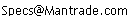Daily Speculations The Web Site of Victor Niederhoffer & Laurel Kenner Dedicated to the scientific method, free markets, deflating ballyhoo, creating value, and laughter;  a forum for us to use our meager abilities to make the world of specinvestments a better place.

Write to us at:(address is not clickable)

 9/25/2005 Rational Trig and Markets, by Doc Alex Castaldo Last week the Bloomberg newswire carried the surprising announcement that Australian mathematician Norman Wildberger has developed a new form of trigonometry that does not use sines, cosines, etc., but can nevertheless solve all trigonometric problems (and do it better, he claims, than the classical trigonometry invented 3,000 years ago). Called Rational Trigonometry, Wildberger's invention does not even use distance and angle, but replaces them with two new notions: "quadrance" and "spread" (see below). I was skeptical, to say the least, but after reading the first chapter of his book, I see nothing obviously wrong or nonsensical in what he is saying. Here is a brief summary: Def. The "quadrance" between two points is the square of the distance, i.e. the quadrance between A_1 = [x_1, y_1] and A_2 = [x_2, y_2] is defined as Q(A_1, A_2) = (x_2 - x_1)^2 + (y_2 - y_1)^2 Def. The "spread" betwen two lines L_1 (with equation a_1*x + b_1*y + c_1 = 0) and L_2 (with equation a_2*x + b_2*y + c_2 = 0) is defined as s(L_1, L_2) = (a_1*b_2 - a_2*b_1)^2 / ((a_1^2 + b_1^2)*(a_2^2 + b_2^2)) The spread is 0 when the lines are parallel, is equal to 1 when the two lines are perpendicular. [The spread is like a sine squared, but remember that we are not supposed to use sines and cosines anymore]. Wildberger claims that all trigonometric problems can be solved by applying what he calls The Five Main Laws of Rational Trigonometry. We are given three distinct points A_1, A_2 and A_3. We use the notation Q_1 = Q(A_2, A_3) etc. and s_1 = s(A_1 A_2, A_1 A_3) etc. The Triple Quad Formula The three points are collinear iff (Q_1 + Q_2 + Q_3)^2 = 2*(Q_1^2 + Q_2^2 + Q_3^2) Pythagoras Theorem The lines A_1 A_3 and A_2 A_3 are perpendicular iff Q_1 + Q_2 = Q_3 [this is familiar] The Spread Law For any triangle with non-zero quadrances s_1/Q_1 = s_2/Q_2 = s_3/Q_3 [This is recognizable as similar to the Sine Law, but with all terms squared] The Cross Law For any triangle (Q_1 + Q_2 - Q_3)^2 = 4 * Q_1 * Q_2 * (1-s_3) The Triple Spread formula For any triangle (s_1 + s_2 + s_3)^2 = 2*(s_1^2 + s_2^2 + s_3^2) + 4*s_1*s_2*s_3 Does it really work? Does it offer any advantage over classical trigonometry? Even if it does, will people want to abandon familiar concepts to learn the new ones? I doubt it. But Manchester Trading will try to be the first to apply these concepts to financial markets.